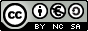Multiples and factors. Prime numbers First and Second Year of Secondary Education

1. Prime factor decomposition of a number.

Prime factor decomposition of a number means writing it as a product of prime factors.
- To factorise a number, divide it by the first possible prime number.
- Take the resulting quotient below the number.
- If it is possible, continue dividing this quotient successively by the same prime number.
- When you cannot do the division by this prime number, divide it by the next possible prime number.
- And so forth until the final quotient is 1.
- Finally write this number as a product of powers of prime factors.

Esta unidad interactiva requiere la máquina virtual de Java J2RE.
 This window works out the prime factor decomposition of a number. In order to do this, type any number in the control box below and hit Enter.  To see how other numbers are factorised, you can change the value of the number by using the up and down triangles. The largest number you can type is a five-digit number.

Investigate:

- Do you think that the size of a number is related to the quantity of prime factors?

- If you work out the prime factor decomposition of a large number, do you expect it to be formed by many factors?

- How many factors compose a prime number?

2. Find the number from which this prime factor decomposition comes.
Esta unidad interactiva requiere la máquina virtual de Java J2RE.
 Work out the product of these powers, type the number and hit Enter. When you click on Init, a random factor decomposition of another number will appear.

 Eduardo Barbero CorralSpanish Ministry of Education. Year 2007